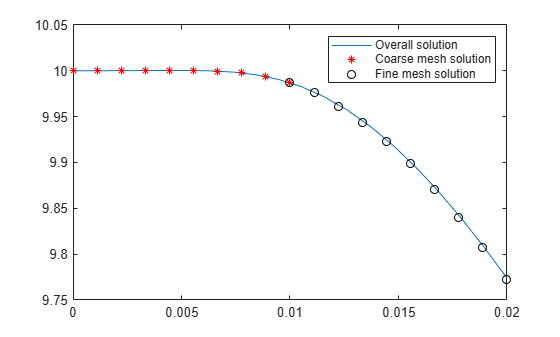# Set Initial Condition for Model with Fine Mesh Using Solution Obtained with Coarser Mesh

Set initial conditions for a model with a fine mesh by using the coarse-mesh solution from a previous analysis.

Create a PDE model and include the geometry of the built-in function `squareg`.

```model = createpde; geometryFromEdges(model,@squareg);```

Specify the coefficients, apply boundary conditions, and set initial conditions.

```specifyCoefficients(model,'m',0,'d',1,'c',5,'a',0,'f',0.1); applyBoundaryCondition(model,'dirichlet','Edge',1,'u',1); setInitialConditions(model,10);```

Generate a comparatively coarse mesh with the target maximum element edge length of 0.1.

`generateMesh(model,'Hmax',0.1);`

Solve the model for the entire time span of 0 through 0.02 seconds.

```tlist = linspace(0,2E-2,20); Rtotal = solvepde(model,tlist);```

Interpolate the solution at the origin for the entire time span.

`singleSpanSol = Rtotal.interpolateSolution(0,0,1:numel(tlist)); `

Now solve the model for the first half of the time span. You will use this solution as an initial condition when solving the model with a finer mesh for the second half of the time span.

```tlist1 = linspace(0,1E-2,10); R1 = solvepde(model,tlist1);```

Create an interpolant to interpolate the initial condition.

```x = model.Mesh.Nodes(1,:)'; y = model.Mesh.Nodes(2,:)'; interpolant = scatteredInterpolant(x,y,R1.NodalSolution(:,end));```

Generate a finer mesh by setting the target maximum element edge length to 0.05.

`generateMesh(model,'Hmax',0.05);`

Use the coarse mesh model results as the initial condition for the model with the finer mesh. For the definition of the `icFcn` function, see Initial Conditions Function.

`setInitialConditions(model,@(region) icFcn(region,interpolant));`

Solve the model for the second half of the time span.

```tlist2 = linspace(1E-2,2E-2,10); R2 = solvepde(model,tlist2);```

Interpolate the solutions at the origin for the first and the second halves of the time span.

```multispanSol1 = R1.interpolateSolution(0,0,1:numel(tlist1)); multispanSol2 = R2.interpolateSolution(0,0,1:numel(tlist2));```

Plot all three solutions at the origin.

```figure plot(tlist,singleSpanSol) hold on plot(tlist1, multispanSol1,'r*') plot(tlist2, multispanSol2,'ko') legend('Overall solution','Coarse mesh solution', 'Fine mesh solution')```### Initial Conditions Function

```function u0 = icFcn(region,interpolant) u0 = interpolant(region.x',region.y'); end```

## Support평가판 신청